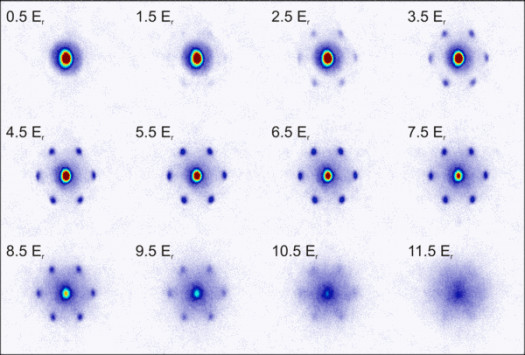# How Well Do You Know Coordinate Plane?

Approved & Edited by ProProfs Editorial Team
At ProProfs Quizzes, our dedicated in-house team of experts takes pride in their work. With a sharp eye for detail, they meticulously review each quiz. This ensures that every quiz, taken by over 100 million users, meets our standards of accuracy, clarity, and engagement.
| Written by Livyn
L
Livyn
Community Contributor
Quizzes Created: 348 | Total Attempts: 140,973
Questions: 10 | Attempts: 152SettingsA coordinate plane is an intersection of two number lines. Just like a regular number line, the ones on a coordinate plane could stretch on infinitely.

• 1.

### A coordinate plane, which is an intersection of how many number lines?

• A.

One

• B.

Two

• C.

Three

• D.

Four

B. Two
Explanation
A coordinate plane is formed by the intersection of two number lines, one horizontal and one vertical. These number lines are called the x-axis and y-axis respectively. The x-axis represents the horizontal values or the values of the first coordinate, while the y-axis represents the vertical values or the values of the second coordinate. Therefore, the correct answer is two.

Rate this question:

• 2.

### The length of a coordinate plane's number lines is known as its ....

• A.

Scale

• B.

Axis

• C.

• D.

Value

A. Scale
Explanation
The length of a coordinate plane's number lines is known as its scale. The scale determines the unit of measurement and helps in understanding the distance between points on the plane. It is used to accurately represent the values and positions of points in the coordinate system.

Rate this question:

• 3.

### By convention, we normally call the horizontal number line the ....

• A.

Y-axis

• B.

A-axis

• C.

X-axis

• D.

Z-axis

C. X-axis
Explanation
The correct answer is x-axis because by convention, the horizontal number line is referred to as the x-axis. This is commonly used in coordinate systems to represent the horizontal position of a point. The x-axis is perpendicular to the y-axis, which represents the vertical position. The z-axis is typically used to represent the depth or height in a three-dimensional coordinate system.

Rate this question:

• 4.

### we normally call the vertical number line the ....

• A.

A-axis

• B.

X-axis

• C.

B-axis

• D.

Y-axis

D. Y-axis
Explanation
The vertical number line is commonly referred to as the y-axis in mathematics. It is used to represent the values of the dependent variable in a coordinate system, while the horizontal number line is called the x-axis. The y-axis is perpendicular to the x-axis and helps in locating points in a two-dimensional plane.

Rate this question:

• 5.

### The axes cut the coordinate plane into how many equal sections or quadrants?

• A.

One

• B.

Two

• C.

Three

• D.

Four

D. Four
Explanation
The axes of the coordinate plane, the x-axis and the y-axis, intersect at the origin and divide the plane into four equal sections or quadrants. Each quadrant is numbered from I to IV, starting from the top right and moving counterclockwise.

Rate this question:

• 6.

### The x-axis is also known as the ....

• A.

Horizontal line

• B.

Vertical line

• C.

Slant line

• D.

None

A. Horizontal line
Explanation
The x-axis is also known as the horizontal line because it represents the horizontal or side-to-side direction on a graph. It is used to plot the independent variable or the variable being measured or observed in an experiment. The y-axis, on the other hand, represents the vertical or up-and-down direction. Together, the x-axis and y-axis create a coordinate system that allows for the graphical representation of data.

Rate this question:

• 7.

### The number line called the y-axis is known as ....

• A.

Horizontal

• B.

Vertical

• C.

Slant

• D.

None

B. Vertical
Explanation
The y-axis on a number line represents the vertical direction. It is used to plot points and measure distances in the vertical plane. The y-axis is perpendicular to the x-axis, which represents the horizontal direction. Therefore, the correct answer is vertical.

Rate this question:

• 8.

### Coordinate Plane is a topic in which course?

• A.

Music

• B.

Sociology

• C.

Mathematics

• D.

French

C. Mathematics
Explanation
The topic of the coordinate plane is a mathematical concept. It is a system used to locate points in a two-dimensional space using a pair of numbers called coordinates. This concept is widely taught in mathematics courses and is an essential foundation for understanding algebra, geometry, and other mathematical topics.

Rate this question:

• 9.

### Who is not a mathematician among them?

• A.

Pythagoras

• B.

Isaac Newton

• C.

Albert Einstein

• D.

Barack Obama

D. Barack Obama
Explanation
Barack Obama is not a mathematician because he is known for his career in politics, specifically as the 44th President of the United States. Pythagoras, Isaac Newton, and Albert Einstein, on the other hand, are renowned mathematicians who have made significant contributions to the field of mathematics.

Rate this question:

• 10.

### Mathematics is usually taught at ....

• A.

School

• B.

Museum

• C.

Church

• D.

MosqueBack to top